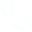# A Complete Guide to Machine Learning Algorithms: Types, How it Works and More

In the world of data science and machine learning, algorithms are everywhere. These specific instructions enable computers to learn new things rather than follow pre-programmed instructions. If you want your computer to be able to identify cats in images or recognize advertisements when listening to the audio, you need algorithms. In this blog post, we will walk through the many different types of machine learning algorithms and briefly introduce how they work. What follows is a series of blog posts on individual ML algorithms and their implementation in Python with case studies from real-world applications.

## How Machine Learning Algorithm Works

A machine learning algorithm is a set of instructions written in code that enables computers to learn from data and make predictions without being programmed to do so. The algorithm is then exposed as a service that enables computers to learn and make predictions. There are two main types of machine learning algorithms: supervised and unsupervised. Supervised learning algorithms are trained with a data set with both an input and an output. The algorithm is trained to find a relationship between input and output data. Unsupervised learning algorithms are trained with data that only has input data and no output data. The algorithm finds patterns in the data and creates new data based on what it finds. Machine learning algorithms use many different techniques to perform their calculations. Depending on the algorithm and data types, many different calculation methods exist.

## Types of Machine Learning Algorithm

### Supervised Machine Learning Algorithms

Supervised learning algorithms require both an input and an expected output. The algorithm is trained with data sets that have both input data and expected output data. The algorithm is then given the data set and input data and is told what the expected output data is. The algorithm makes predictions based on the input data and then is given the expected output data. If the algorithm is accurate, then the algorithm is given a certain score. Supervised learning algorithms are used for classification and regression problems. Classification problems are when a computer is trained to predict a categorical label. Regression problems are when a computer is trained to predict a continuous output.

### Unsupervised Machine Learning Algorithms

Unsupervised learning algorithms are trained with data sets that only have input data without expected output data. The algorithm looks for patterns within the input data and creates new data based on what it sees. There are two types of unsupervised algorithms: clustering algorithms and association rule mining algorithms. Clustering algorithms are trained with data sets that have similar data points together. The algorithm then creates new data points to represent those clusters. Association rule mining algorithms are trained with data sets that have items that frequently appear together. The algorithm finds rules that are associated with those items.

### Reinforcement Machine Learning Algorithms

Reinforcement learning algorithms are trained with data sets that include input data, expected output data, and rewards. The algorithm is given input and expected output data set. The algorithm is also given a reward that is used to measure accuracy. This algorithm is used when there is no correct answer. Instead, the algorithm is given a reward for predicting correctly. The algorithm is retrained with every new data set to improve accuracy.

### Classifier ML Algorithms

Classifier algorithms are used for supervised machine learning algorithms. The algorithm is trained with a data set that has input data, expected output data, and other data sets that are used to validate the accuracy of the algorithm. The algorithm is trained to make predictions based on the input data. The other data sets are used to validate the accuracy of the algorithm. If the algorithm is accurate, the algorithm is used.

## Conclusion

This article discussed the different types of machine learning algorithms, how they work, and what they are used for. We hope that this article has helped you to understand better the algorithms that are used in the field of machine learning.

## Related Articles

• ### A detailed explanation of Hadoop core architecture HDFS

Knowledge Base Team

• ### What Does IOT Mean

Knowledge Base Team

• ### 6 Optional Technologies for Data Storage

Knowledge Base Team

• ### What Is Blockchain Technology

Knowledge Base Team

## Explore More Special Offers

1. ### Short Message Service(SMS) & Mail Service

50,000 email package starts as low as USD 1.99, 120 short messages start at only USD 1.00Contact Us